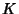## Singular Point (Algebraic Curve)

A singular point of an Algebraic Curve is a point where the curve has nasty'' behavior such as a Cusp or a point of self-intersection (when the underlying fieldis taken as the Reals). More formally, a pointon a curveis singular if theandPartial Derivatives ofare both zero at the point. (If the fieldis not the Reals or Complex Numbers, then the Partial Derivative is computed formally using the usual rules of Calculus.)

Consider the following two examples. For the curvethe Cusp at (0, 0) is a singular point. For the curveis a nonsingular point and this curve is nonsingular.